# Time Series Forecasting Using Deep Learning

This example shows how to forecast time series data using a long short-term memory (LSTM) network.

An LSTM network is a recurrent neural network (RNN) that processes input data by looping over time steps and updating the network state. The network state contains information remembered over all previous time steps. You can use an LSTM network to forecast subsequent values of a time series or sequence using previous time steps as input. To train an LSTM network for time series forecasting, train a regression LSTM network with sequence output, where the responses (targets) are the training sequences with values shifted by one time step. In other words, at each time step of the input sequence, the LSTM network learns to predict the value of the next time step.

There are two methods of forecasting: open loop and closed loop forecasting.

• Open loop forecasting predicts the next time step in a sequence using only the input data. When making predictions for subsequent time steps, you collect the true values from your data source and use those as input. For example, say you want to predict the value for time step $t$ of a sequence using data collected in time steps 1 through $t-1$. To make predictions for time step $t+1$, wait until you record the true value for time step $t$ and use that as input to make the next prediction. Use open loop forecasting when you have true values to provide to the network before making the next prediction.

• Closed loop forecasting predicts subsequent time steps in a sequence by using the previous predictions as input. In this case, the model does not require the true values to make the prediction. For example, say you want to predict the values for time steps $t$ through $t+k$ of the sequence using data collected in time steps 1 through $t-1$ only. To make predictions for time step $i$, use the predicted value for time step $i-1$ as input. Use closed loop forecasting to forecast multiple subsequent time steps or when you do not have the true values to provide to the network before making the next prediction.

This figure shows an example sequence with forecasted values using closed loop prediction.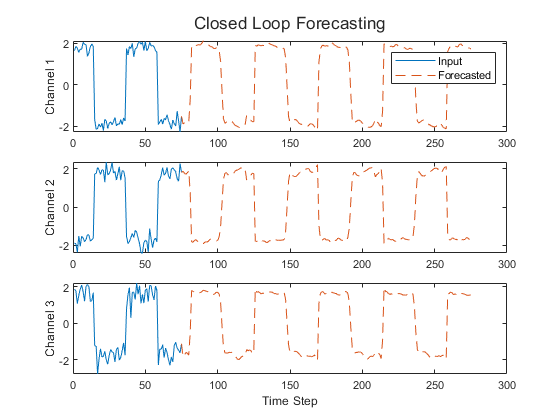This example uses the Waveform data set, which contains 2000 synthetically generated waveforms of varying lengths with three channels. The example trains an LSTM network to forecast future values of the waveforms given the values from previous time steps using both closed loop and open loop forecasting.

Load the example data from `WaveformData.mat`. The data is a `numObservations`-by-1 cell array of sequences, where `numObservations` is the number of sequences. Each sequence is a `numChannels`-by`-numTimeSteps` numeric array, where `numChannels` is the number of channels of the sequence and `numTimeSteps` is the number of time steps of the sequence.

`load WaveformData`

View the sizes of the first few sequences.

`data(1:5)`
```ans=5×1 cell array {3×103 double} {3×136 double} {3×140 double} {3×124 double} {3×127 double} ```

View the number of channels. To train the network, each sequence must have the same number of channels.

`numChannels = size(data{1},1)`
```numChannels = 3 ```

Visualize the first few sequences in a plot.

```figure tiledlayout(2,2) for i = 1:4 nexttile stackedplot(data{i}') xlabel("Time Step") end```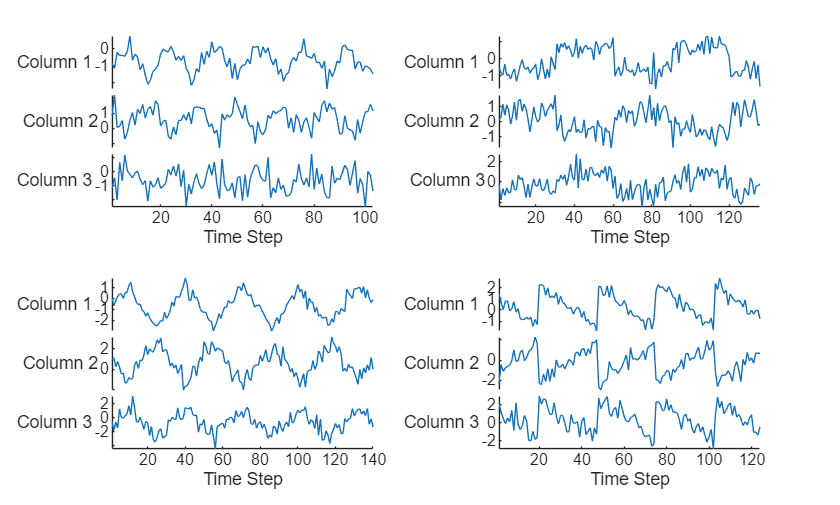Partition the data into training and test sets. Use 90% of the observations for training and the remainder for testing.

```numObservations = numel(data); idxTrain = 1:floor(0.9*numObservations); idxTest = floor(0.9*numObservations)+1:numObservations; dataTrain = data(idxTrain); dataTest = data(idxTest);```

### Prepare Data for Training

To forecast the values of future time steps of a sequence, specify the targets as the training sequences with values shifted by one time step. In other words, at each time step of the input sequence, the LSTM network learns to predict the value of the next time step. The predictors are the training sequences without the final time step.

```for n = 1:numel(dataTrain) X = dataTrain{n}; XTrain{n} = X(:,1:end-1); TTrain{n} = X(:,2:end); end```

For a better fit and to prevent the training from diverging, normalize the predictors and targets to have zero mean and unit variance. When you make predictions, you must also normalize the test data using the same statistics as the training data. To easily calculate the mean and standard deviation over all sequences, concatenate the sequences in the time dimension.

```muX = mean(cat(2,XTrain{:}),2); sigmaX = std(cat(2,XTrain{:}),0,2); muT = mean(cat(2,TTrain{:}),2); sigmaT = std(cat(2,TTrain{:}),0,2); for n = 1:numel(XTrain) XTrain{n} = (XTrain{n} - muX) ./ sigmaX; TTrain{n} = (TTrain{n} - muT) ./ sigmaT; end```

### Define LSTM Network Architecture

Create an LSTM regression network.

• Use a sequence input layer with an input size that matches the number of channels of the input data.

• Use an LSTM layer with 128 hidden units. The number of hidden units determines how much information is learned by the layer. Using more hidden units can yield more accurate results but can be more likely to lead to overfitting to the training data.

• To output sequences with the same number of channels as the input data, include a fully connected layer with an output size that matches the number of channels of the input data.

• Finally, include a regression layer.

```layers = [ sequenceInputLayer(numChannels) lstmLayer(128) fullyConnectedLayer(numChannels) regressionLayer];```

### Specify Training Options

Specify the training options.

• Train for 200 epochs. For larger data sets, you might not need to train for as many epochs for a good fit.

• In each mini-batch, left-pad the sequences so they have the same length. Left-padding prevents the network from predicting padding values at the ends of sequences.

• Shuffle the data every epoch.

• Display the training progress in a plot.

• Disable the verbose output.

```options = trainingOptions("adam", ... MaxEpochs=200, ... SequencePaddingDirection="left", ... Shuffle="every-epoch", ... Plots="training-progress", ... Verbose=0);```

### Train Neural Network

Train the LSTM network with the specified training options using the `trainNetwork` function.

`net = trainNetwork(XTrain,TTrain,layers,options);`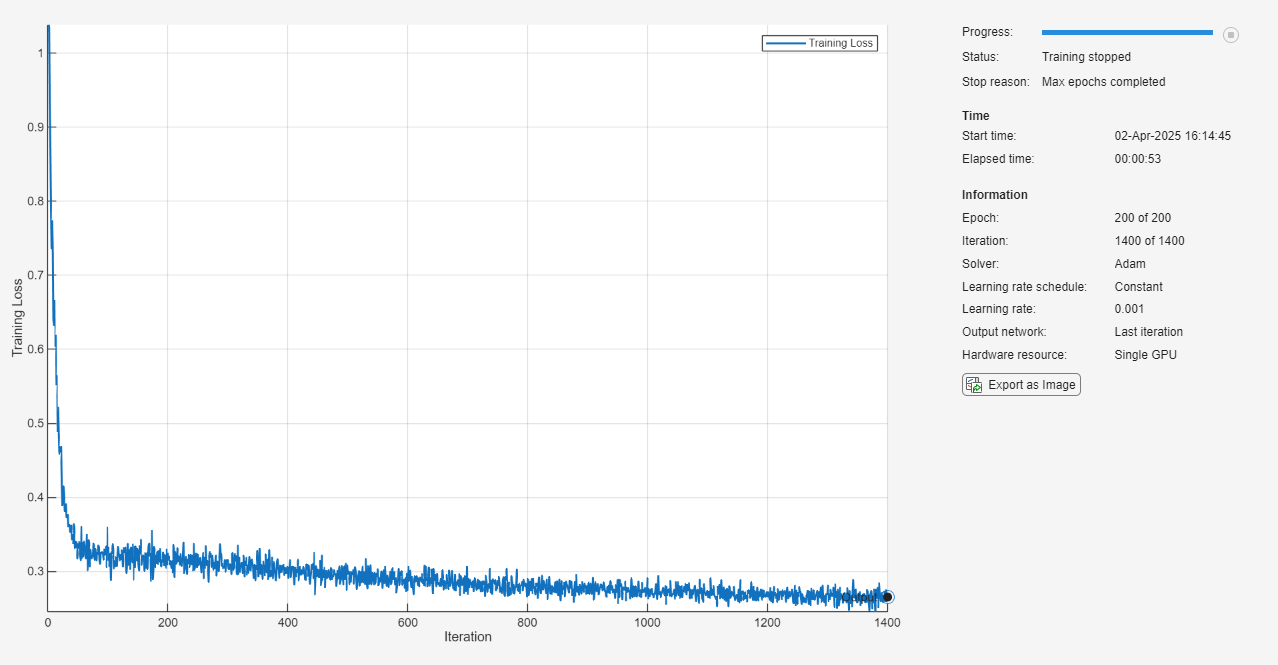### Test Network

Prepare the test data for prediction using the same steps as for the training data.

Normalize the test data using the statistics calculated from the training data. Specify the targets as the test sequences with values shifted by one time step and the predictors as the test sequences without the final time step.

```for n = 1:size(dataTest,1) X = dataTest{n}; XTest{n} = (X(:,1:end-1) - muX) ./ sigmaX; TTest{n} = (X(:,2:end) - muT) ./ sigmaT; end```

Make predictions using the test data. Specify the same padding options as for training.

`YTest = predict(net,XTest,SequencePaddingDirection="left");`

To evaluate the accuracy, for each test sequence, calculate the root mean squared error (RMSE) between the predictions and the target.

```for i = 1:size(YTest,1) rmse(i) = sqrt(mean((YTest{i} - TTest{i}).^2,"all")); end```

Visualize the errors in a histogram. Lower values indicate greater accuracy.

```figure histogram(rmse) xlabel("RMSE") ylabel("Frequency")```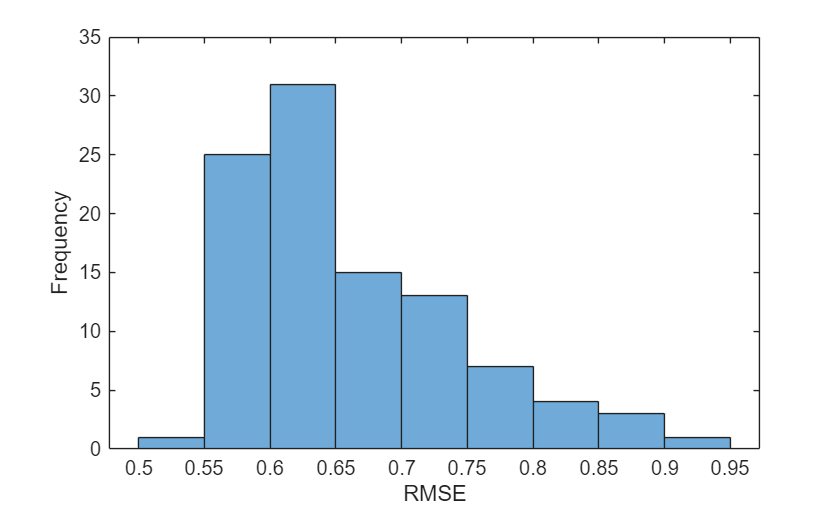Calculate the mean RMSE over all test observations.

`mean(rmse)`
```ans = single 0.5080 ```

### Forecast Future Time Steps

Given an input time series or sequence, to forecast the values of multiple future time steps, use the `predictAndUpdateState` function to predict time steps one at a time and update the network state at each prediction. For each prediction, use the previous prediction as the input to the function.

Visualize one of the test sequences in a plot.

```idx = 2; X = XTest{idx}; T = TTest{idx}; figure stackedplot(X',DisplayLabels="Channel " + (1:numChannels)) xlabel("Time Step") title("Test Observation " + idx)```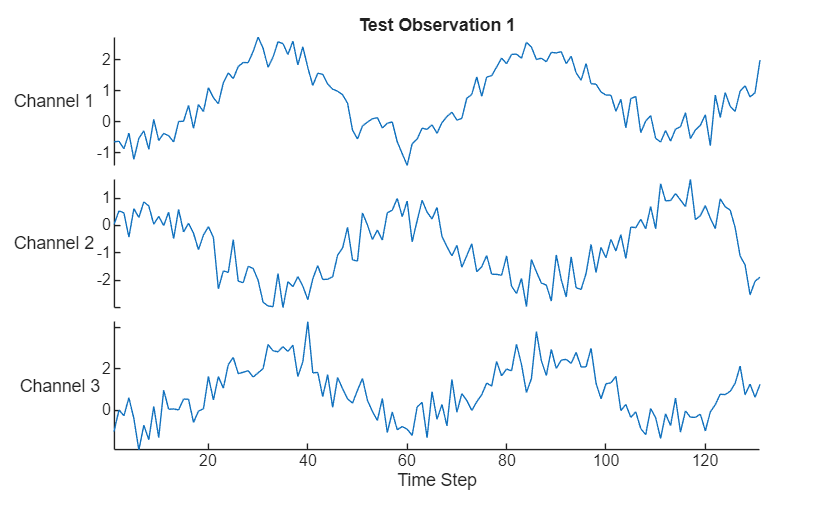#### Open Loop Forecasting

Open loop forecasting predicts the next time step in a sequence using only the input data. When making predictions for subsequent time steps, you collect the true values from your data source and use those as input. For example, say you want to predict the value for time step $t$ of a sequence using data collected in time steps 1 through $t-1$. To make predictions for time step $t+1$, wait until you record the true value for time step $t$ and use that as input to make the next prediction. Use open loop forecasting when you have true values to provide to the network before making the next prediction.

Initialize the network state by first resetting the state using the `resetState` function, then make an initial prediction using the first few time steps of the input data. Update the network state using the first 75 time steps of the input data.

```net = resetState(net); offset = 75; [net,~] = predictAndUpdateState(net,X(:,1:offset));```

To forecast further predictions, loop over time steps and update the network state using the `predictAndUpdateState` function. Forecast values for the remaining time steps of the test observation by looping over the time steps of the input data and using them as input to the network. The first prediction is the value corresponding to the time step `offset + 1`.

```numTimeSteps = size(X,2); numPredictionTimeSteps = numTimeSteps - offset; Y = zeros(numChannels,numPredictionTimeSteps); for t = 1:numPredictionTimeSteps Xt = X(:,offset+t); [net,Y(:,t)] = predictAndUpdateState(net,Xt); end```

Compare the predictions with the target values.

```figure t = tiledlayout(numChannels,1); title(t,"Open Loop Forecasting") for i = 1:numChannels nexttile plot(T(i,:)) hold on plot(offset:numTimeSteps,[T(i,offset) Y(i,:)],'--') ylabel("Channel " + i) end xlabel("Time Step") nexttile(1) legend(["Input" "Forecasted"])```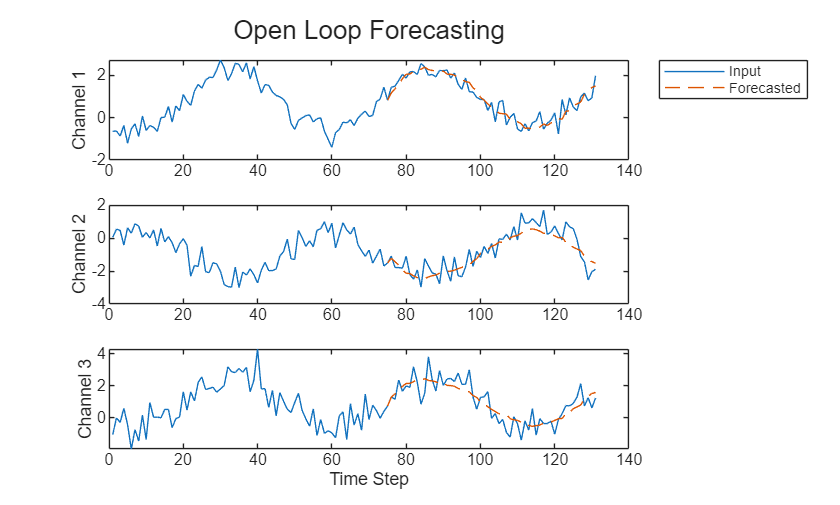#### Closed Loop Forecasting

Closed loop forecasting predicts subsequent time steps in a sequence by using the previous predictions as input. In this case, the model does not require the true values to make the prediction. For example, say you want to predict the value for time steps $t$ through $t+k$ of the sequence using data collected in time steps 1 through $t-1$ only. To make predictions for time step $i$, use the predicted value for time step $i-1$ as input. Use closed loop forecasting to forecast multiple subsequent time steps or when you do not have true values to provide to the network before making the next prediction.

Initialize the network state by first resetting the state using the `resetState` function, then make an initial prediction `Z` using the first few time steps of the input data. Update the network state using the first 75 time steps of the input data.

```net = resetState(net); offset = size(X,2); [net,Z] = predictAndUpdateState(net,X);```

To forecast further predictions, loop over time steps and update the network state using the `predictAndUpdateState` function. Forecast the next 200 time steps by iteratively passing the previous predicted value to the network. Because the network does not require the input data to make any further predictions, you can specify any number of time steps to forecast.

```numPredictionTimeSteps = 200; Xt = Z(:,end); Y = zeros(numChannels,numPredictionTimeSteps); for t = 1:numPredictionTimeSteps [net,Y(:,t)] = predictAndUpdateState(net,Xt); Xt = Y(:,t); end```

Visualize the forecasted values in a plot.

```numTimeSteps = offset + numPredictionTimeSteps; figure t = tiledlayout(numChannels,1); title(t,"Closed Loop Forecasting") for i = 1:numChannels nexttile plot(T(i,1:offset)) hold on plot(offset:numTimeSteps,[T(i,offset) Y(i,:)],'--') ylabel("Channel " + i) end xlabel("Time Step") nexttile(1) legend(["Input" "Forecasted"])```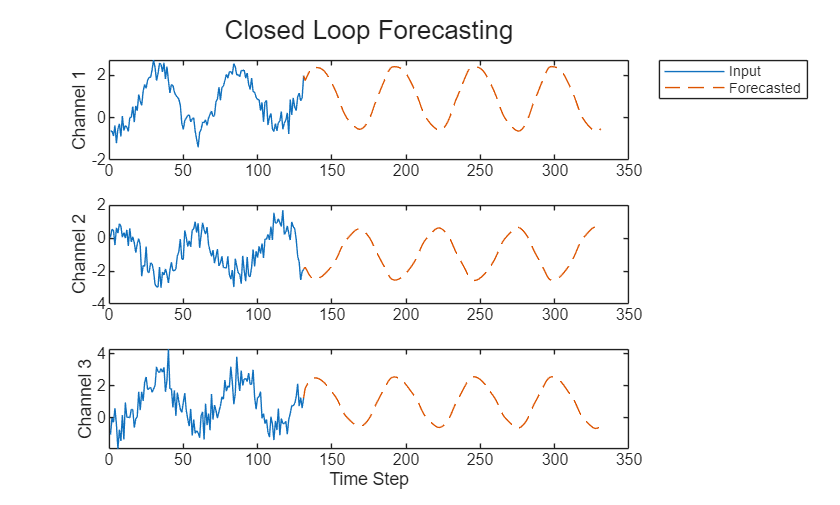Closed loop forecasting allows you to forecast an arbitrary number of time steps, but can be less accurate when compared to open loop forecasting because the network does not have access to the true values during the forecasting process.